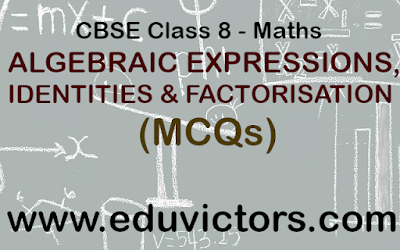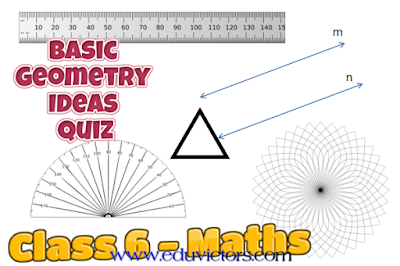## Pages

Showing posts with label maths-quiz. Show all posts
Showing posts with label maths-quiz. Show all posts

## ALGEBRAIC EXPRESSIONS, IDENTITIES AND FACTORISATION

(MCQs)

Q1: Which is the like term as 24a²bc?
(a) 13 × 8a × 2b × c × a
(b) 8 × 3 × a × b × c
(c) 3 × 8 × a × b × c × c
(d) 3 × 8 × a × b × b × c## Basic Geometry Ideas - Quiz

Class 6 - Mathematics

Q1: Three or more lines which pass through same point are called

(a) intersecting lines
(b) parallel lines
(c) perpendicular lines
(d) concurrent lines.

Q2: Geometrical figure which has no dimension is

(a) line
(b) plane
(c) line segment
(d) point.

Q3: The lines which do not intersect and have equal distance between them are called:

(a) parallel lines
(b) perpendicular lines
(c) intersecting lines
(d) straight lines